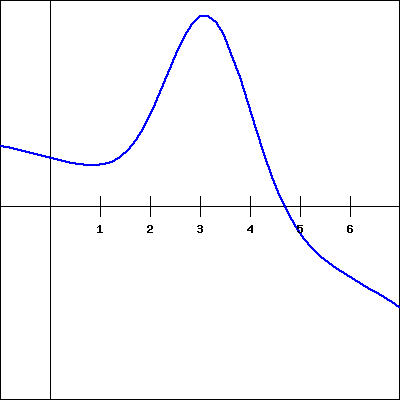For the function $f(x)$ shown in the graph below, sketch a graph of the derivative. You will then be picking which of the following is the correct derivative graph, but should be sure to first sketch the derivative yourself.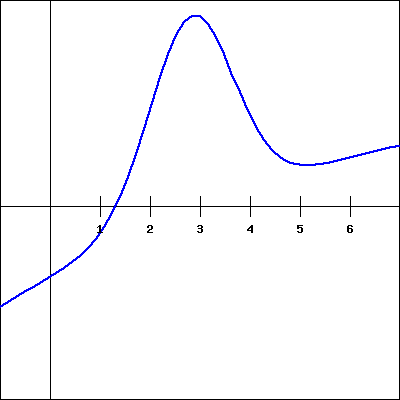Which of the following graphs is the derivative of $f(x)$?

(Click on a graph to enlarge it.)

 1.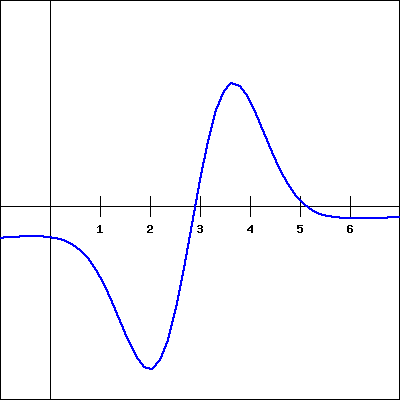2.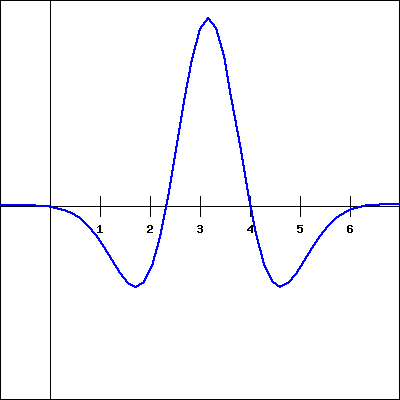3.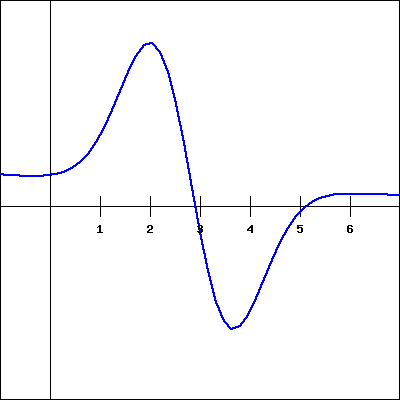4.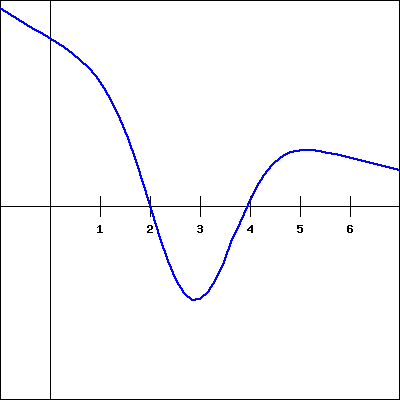5.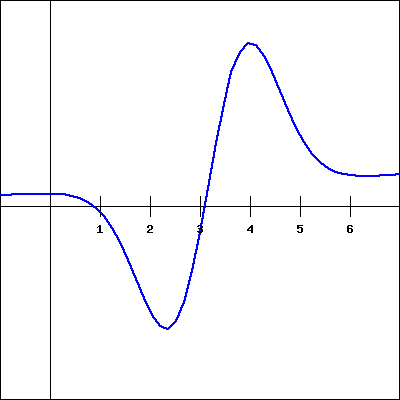6.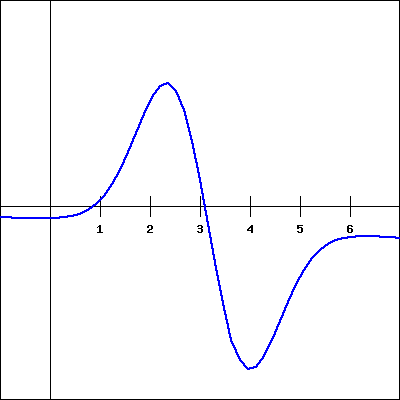7.8.